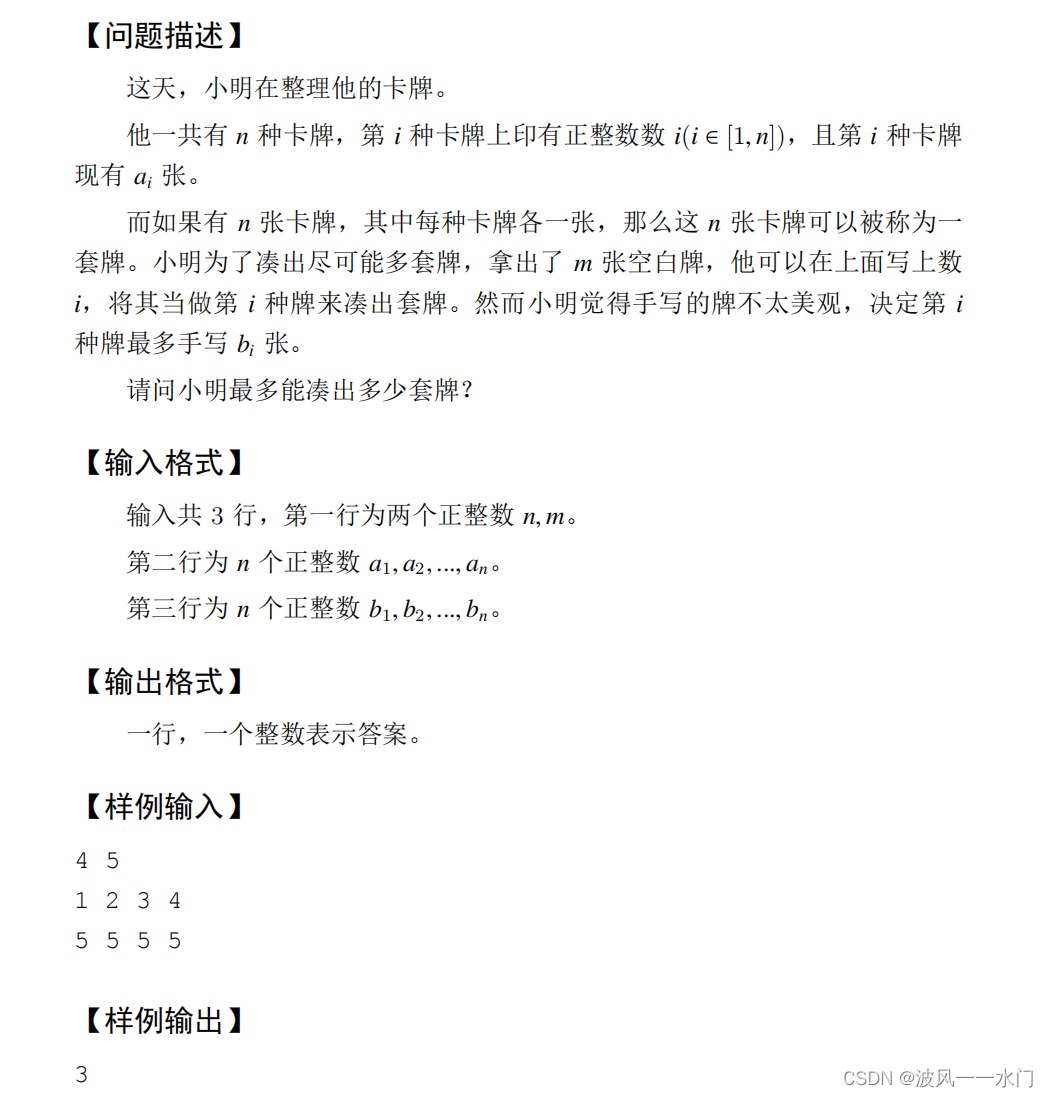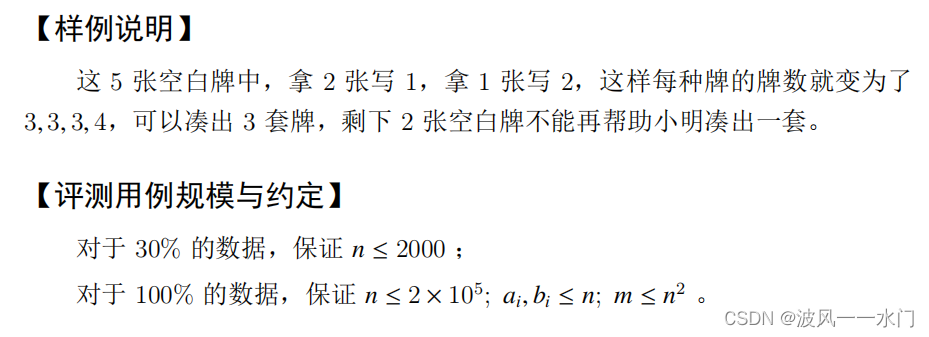## ACM集训7月4号_波风一一水门的博客-程序员宅基地

1、二分
2、前缀和与差分
3、STL
4、双指针
5、离散化
6、位运算

### 二分#include <bits/stdc++.h>
using namespace std;
#define int long long
const int N = 1e5 + 10;

int n, m;
int a[N], b[N];

bool check(int x)
{

int ans = 0;
for(int i = 1; i <= n; i ++ )
{

if(a[i] < x )
{

if(b[i] < x - a[i]) return false;
ans += x - a[i];
}
}
if(ans > m) return false;
return true;
}

signed main()
{

cin >> n >> m;
for(int i = 1; i <= n; i ++ ) cin >> a[i];
for(int i = 1; i <= n; i ++ ) cin >> b[i];
int l = 0, r = n * 2;
while(l < r)
{

int mid = (l + r + 1) / 2;
if(check(mid)) l = mid;
else r = mid - 1;
}
cout << l << endl;
}


### 一维前缀和与一维差分

sum[i] = sum[i - 1] + a[i]
sum[r] - sum[l - 1]


b[i] = a[i] - a[i - 1]
b[l] += k, b[r + 1] -= k


### 二维前缀和与二维差分

sum[i][j] = sum[i - 1][j] + sum[i][j - 1] - sum[i - 1][j - 1] + a[i][j]


sum[x2][y2] - sum[x2][y1 - 1] - sum[x1 - 1][y2] + sum[x1 - 1][y1 - 1]


b[i][j] = a[i][j] - a[i - 1][j] - a[i][j - 1] + a[i - 1][j - 1]


b[x1][y1] += k , b[x1][y2 + 1] -= k, b[x2 + 1][y1] -= k, b[x2 + 1][y2 + 1] += k


### STL

stack：top
queue：front，back
priority_queue：top

vector，map，哈希表，set通用方法：size，clear，erase，begin，end

vector：push_back，pop_back，rbegin，rend
map：count，insert ，lower_bound，upper_bound
unordered_map
set：lower_bound upper_bound

### 双指针

#include <bits/stdc++.h>
using namespace std;
const int N = 1e5 + 10;
// 维护一个合法的 [j , i] 的区间，保证区间内所有元素不重复
int a[N];
int st[N]; // 记录 [j , i] 区间内元素出现次数

int main()
{

int n;
cin >> n;
for(int i = 1; i <= n; i ++ ) cin >> a[i];
int ans = 0;
for(int i = 1, j = 1; i <= n; i ++ ) //每次将右区间扩大 1， 同时更新 st 数组使 st[i]++，根据新添加的元素 a[i] 判断 st[a[R]] 是否 > 1
{

st[a[i]] ++ ;
while(st[a[i]] > 1)//持续扩大左区间，同时更新 st 数组使 st[j] -- ，直到 st[i] == 1，说明找到合法区间
{

st[a[j]] -- ;
j ++ ;
}
ans = max(ans, i - j + 1);
}
cout << ans << endl;
}


### 离散化

#include <bits/stdc++.h>
using namespace std;
const int N = 1e5 + 10, INF = 1e9 + 10;
typedef long long LL;
typedef pair<int, int> PII;

vector<PII> a;
map<int, int> mp;

int main()
{

int n, m;
cin >> n >> m;
while(n --)
{

int x, c;
cin >> x >> c;
mp[x] += c;
}
int sum = 0;
for(auto x : mp)
{

a.push_back({
x.first, sum});  //这里的sum不包含x.first上的值，方便使用upper_bound()
sum += x.second;
}
a.push_back({
INF, sum});  //最后加一个无穷大的点，方便处理
while(m --)
{

int l, r;
cin >> l >> r;
auto p1 = upper_bound(a.begin(), a.end(), (PII){
l , -INF}); //找到第一个大于等于l的点
auto p2 = upper_bound(a.begin(), a.end(), (PII){
r , INF});  //找到第一个大于r的点
cout << p2 -> second - p1 -> second << endl;
}
return 0;
}


### 位运算lowbit定义：

lowbit 实现：

int lowbit(int x)
{

return x & -x;
}


#include <bits/stdc++.h>
using namespace std;
const int N = 1e5 + 10;

int lowbit(int x)
{

return x & -x;
}

int main()
{

int n;
cin >> n;
for(int i = 1; i <= n; i ++ )
{

int x, ans = 0;
cin >> x;
while(x)
{

x -= lowbit(x);
ans ++ ;
}
cout << ans << " ";
}
}


## codeforce讲题

#### Required Length

x x 更新过程：2，4，16，96，576或864
bfs直接模拟过程，同时标记已经进入过队列的值，保证不会有点重复进队，第一个位数到达 n 的值的深度就是答案，类似于最短路

#include <bits/stdc++.h>
using namespace std;
#define int long long
const int N = 1e5 + 10;
typedef pair<int, int> PII;

int n, x;
// unordered_map<int, bool> mp; 注意：用c++20交会T，因为数据卡c++20的哈希表，建议用稳定查询logn的map
map<int, int> mp;

int size(int x)
{

int cnt = 0;
while(x)
{

x /= 10;
cnt ++ ;
}
return cnt;
}

int bfs()
{

queue<PII> q;
q.push({
x, 1});
mp[x] = true;
while(q.size())
{

PII t = q.front();
q.pop();
if(size(t.first) == n) return t.second;
int tmp = t.first;
while(tmp)
{

int y = t.first * (tmp % 10);
if(!mp.count(y)) q.push({
y, t.second + 1});
mp[y] = true;
tmp /= 10;
}
}
return 0;
}

signed main()
{

cin >> n >> x;
cout << bfs() - 1 << endl;
}


#### Where is the Pizza?

8
1 6 4 7 2 3 8 5
3 2 8 1 4 5 6 7
1 0 0 7 0 3 0 5

#include <bits/stdc++.h>
using namespace std;
#define int long long
const int N = 1e5 + 10, mod = 1e9 + 7;
typedef pair<int, int> PII;

int n;
int a[N], b[N], c[N], g[N];

void init(int n)
{

for(int i = 1; i <= n; i ++ ) g[i] = i;
}

int find(int x)
{

if(x != g[x]) g[x] = find(g[x]);
return g[x];
}

signed main()
{

int t;
cin >> t;
while(t -- )
{

int n;
cin >> n;
init(n);
for(int i = 1; i <= n; i ++ ) cin >> a[i];
for(int i = 1; i <= n; i ++ ) cin >> b[i];
for(int i = 1; i <= n; i ++ ) cin >> c[i];
int ans = 1;
for(int i = 1; i <= n; i ++ )
{

if(c[i] == 0 && a[i] != b[i])
{

int ga = find(a[i]);
int gb = find(b[i]);
if(ga == gb) ans = ans * 2 % mod;
else g[gb] = ga;
}
}
cout << ans << endl;
}
}


#### Tokitsukaze and Good 01-String (hard version)

00001000
01 不合法，因为前后合法子串都是00，所以应该把 1 变成 0，这样才是要求的最小段数

#include <bits/stdc++.h>
using namespace std;
const int N = 1e5 + 10;

vector<int> h;

int main()
{

int t;
cin >> t;
while(t -- )
{

int n;
string a;
cin >> n >> a;
h.clear();
int ans = 0, res = 1;
for(int i = 0; i < n; i += 2)
{

if(a[i] != a[i + 1]) ans ++ ;
else h.push_back(a[i]);
}
for(int i = 1; i < h.size(); i ++ )
{

if(h[i] != h[i - 1]) res ++ ;
}
cout << ans << " " << res << endl;
}
}


### myeclipse安装-每一台电脑都有各自的难言之隐_java.version=20.0.1 java.vendor=oracle corporation-程序员宅基地

java版本是1.8的myeclipse 2017好久没用，然后破解好的就直接就失效了，想着反正版本不是最新的，下个更新的试试。然后就把17的卸了换19的，短短两年就换新，十有八九是要出事的。然后 myeclipse 2019无法打开现在先不慌，打开它给的这个文件看看。!SESSION 2020-03-16 20:22:52.984 ---------------..._java.version=20.0.1 java.vendor=oracle corporation bootloader constants: os=

### js写别踩白块游戏_js脚踩白块怎么用js写出方格_源@的博客-程序员宅基地

<!DOCTYPE HTML><html lang = 'en'> <head> <meta charset = 'UTF-8'/> <title>Document</title> <style> *{ padding:0; margin:0; } .boundary{ position:relative; overflow:hidden; width_js脚踩白块怎么用js写出方格